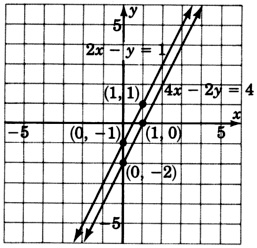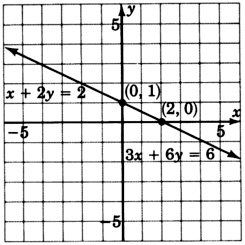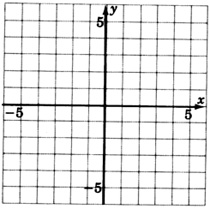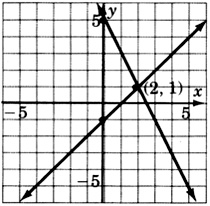# 11.2 Elimination by substitution  (Page 2/2)

 Page 2 / 2

## Substitution and parallel lines

If computations eliminate all the variables and produce a contradiction, the two lines of a system are parallel, and the system is called inconsistent.

## Sample set b

Solve the system $\begin{array}{lll}\left\{\begin{array}{l}2x-y=1\\ 4x-2y=4\end{array}\hfill & \hfill & \begin{array}{l}\left(1\right)\\ \left(2\right)\end{array}\hfill \end{array}$

Step 1:  Solve equation 1 for $y.$
$\begin{array}{lll}\hfill 2x-y& =\hfill & 1\hfill \\ \hfill -y& =\hfill & -2x+1\hfill \\ \hfill y& =\hfill & 2x-1\hfill \end{array}$

Step 2:  Substitute the expression $2x-1$ for $y$ into equation 2.
$4x-2\left(2x-1\right)=4$

Step 3:  Solve the equation obtained in step 2.
$\begin{array}{lll}4x-2\left(2x-1\right)\hfill & =\hfill & 4\hfill \\ \hfill 4x-4x+2& =\hfill & 4\hfill \\ \hfill 2& \ne \hfill & 4\hfill \end{array}$

Computations have eliminated all the variables and produce a contradiction. These lines are parallel.This system is inconsistent.

## Practice set b

Slove the system $\left\{\begin{array}{l}\text{\hspace{0.17em}}\text{\hspace{0.17em}}7x-3y=2\\ 14x-6y=1\end{array}$

Substitution produces $4\ne 1,$ or $\frac{1}{2}\ne 2$ , a contradiction. These lines are parallel and the system is inconsistent.

## Substitution and coincident lines

The following rule alerts us to the fact that the two lines of a system are coincident.

## Substitution and coincident lines

If computations eliminate all the variables and produce an identity, the two lines of a system are coincident and the system is called dependent.

## Sample set c

Solve the system $\begin{array}{lll}\left\{\begin{array}{l}4x+8y=8\\ 3x+6y=6\end{array}\hfill & \hfill & \begin{array}{l}\left(1\right)\\ \left(2\right)\end{array}\hfill \end{array}$

Step 1:  Divide equation 1 by 4 and solve for $x.$
$\begin{array}{lll}\hfill 4x+8y& =\hfill & 8\hfill \\ \hfill x+2y& =\hfill & 2\hfill \\ \hfill x& =\hfill & -2y+2\hfill \end{array}$

Step 2:  Substitute the expression $-2y+2$ for $x$ in equation 2.
$3\left(-2y+2\right)+6y=6$

Step 3:  Solve the equation obtained in step 2.
$\begin{array}{lll}\hfill 3\left(-2y+2\right)+6y& =\hfill & 6\hfill \\ \hfill -6y+6+6y& =\hfill & 6\hfill \\ \hfill 6& =\hfill & 6\hfill \end{array}$

Computations have eliminated all the variables and produced an identity. These lines are coincident.This system is dependent.

## Practice set c

Solve the system $\left\{\begin{array}{l}\text{\hspace{0.17em}}\text{\hspace{0.17em}}4x+3y=\text{\hspace{0.17em}}\text{\hspace{0.17em}}\text{\hspace{0.17em}}\text{\hspace{0.17em}}1\\ -8x-6y=-2\end{array}$

Computations produce $-2=-2,$ an identity. These lines are coincident and the system is dependent.

Systems in which a coefficient of one of the variables is not 1 or cannot be made to be 1 without introducing fractions are not well suited for the substitution method. The problem in Sample Set D illustrates this “messy” situation.

## Sample set d

Solve the system $\begin{array}{lll}\left\{\begin{array}{l}3x+2y=1\\ 4x-3y=3\end{array}\hfill & \hfill & \begin{array}{l}\left(1\right)\\ \left(2\right)\end{array}\hfill \end{array}$

Step 1:  We will solve equation $\left(1\right)$ for $y.$
$\begin{array}{lll}\hfill 3x+2y& =\hfill & 1\hfill \\ \hfill 2y& =\hfill & -3x+1\hfill \\ \hfill y& =\hfill & \frac{-3}{2}x+\frac{1}{2}\hfill \end{array}$

Step 2:  Substitute the expression $\frac{-3}{2}x+\frac{1}{2}$ for $y$ in equation $\left(2\right).$
$4x-3\left(\frac{-3}{2}x+\frac{1}{2}\right)=3$

Step 3:  Solve the equation obtained in step 2.
$\begin{array}{lllll}\hfill 4x-3\left(\frac{-3}{2}x+\frac{1}{2}\right)& =\hfill & 3\hfill & \hfill & \text{Multiply\hspace{0.17em}both\hspace{0.17em}sides\hspace{0.17em}by\hspace{0.17em}the\hspace{0.17em}LCD},\text{\hspace{0.17em}2}.\hfill \\ \hfill 4x+\frac{9}{2}x-\frac{3}{2}& =\hfill & 3\hfill & \hfill & \hfill \\ \hfill 8x+9x-3& =\hfill & 6\hfill & \hfill & \hfill \\ \hfill 17x-3& =\hfill & 6\hfill & \hfill & \hfill \\ \hfill 17x& =\hfill & 9\hfill & \hfill & \hfill \\ \hfill x& =\hfill & \frac{9}{17}\hfill & \hfill & \hfill \end{array}$

Step 4:  Substitute $x=\frac{9}{17}$ into the equation obtained in step $1,\text{\hspace{0.17em}}y=\frac{-3}{2}x+\frac{1}{2}.$
$y=\frac{-3}{2}\left(\frac{9}{17}\right)+\frac{1}{2}$
$y=\frac{-27}{34}+\frac{17}{34}=\frac{-10}{34}=\frac{-5}{17}$
We now have $x=\frac{9}{17}$ and $y=\frac{-5}{17}.$

Step 5:  Substitution will show that these values of $x$ and $y$ check.

Step 6:  The solution is $\left(\frac{9}{17},\frac{-5}{17}\right).$

## Practice set d

Solve the system $\left\{\begin{array}{l}9x-5y=-4\\ 2x+7y=-9\end{array}$

These lines intersect at the point $\left(-1,-1\right).$

## Exercises

For the following problems, solve the systems by substitution.

$\left\{\begin{array}{lll}3x+2y\hfill & =\hfill & 9\hfill \\ \text{\hspace{0.17em}}\text{\hspace{0.17em}}\text{\hspace{0.17em}}\text{\hspace{0.17em}}\text{\hspace{0.17em}}\text{\hspace{0.17em}}\text{\hspace{0.17em}}\text{\hspace{0.17em}}y\hfill & =\hfill & -3x+6\hfill \end{array}$

$\left(1,3\right)$

$\left\{\begin{array}{lll}5x-3y\hfill & =\hfill & -6\hfill \\ \text{\hspace{0.17em}}\text{\hspace{0.17em}}\text{\hspace{0.17em}}\text{\hspace{0.17em}}\text{\hspace{0.17em}}\text{\hspace{0.17em}}\text{\hspace{0.17em}}\text{\hspace{0.17em}}y\hfill & =\hfill & -4x+12\hfill \end{array}$

$\left\{\begin{array}{lll}2x+2y\hfill & =\hfill & 0\hfill \\ \text{\hspace{0.17em}}\text{\hspace{0.17em}}x\hfill & =\hfill & 3y-4\hfill \end{array}$

$\left(-1,1\right)$

$\left\{\begin{array}{lll}3x+5y\hfill & =\hfill & 9\hfill \\ \text{\hspace{0.17em}}x\hfill & =\hfill & 4y-14\hfill \end{array}$

$\left\{\begin{array}{lll}-3x+y\hfill & =\hfill & -4\hfill \\ 2x+3y\hfill & =\hfill & 10\hfill \end{array}$

$\left(2,2\right)$

$\left\{\begin{array}{lll}-4x+y\hfill & =\hfill & -7\hfill \\ 2x+5y\hfill & =\hfill & 9\hfill \end{array}$

$\left\{\begin{array}{lll}6x-6\hfill & =\hfill & 18\hfill \\ x+3y\hfill & =\hfill & 3\hfill \end{array}$

$\left(4,-\frac{1}{3}\right)$

$\left\{\begin{array}{lll}-x-y\hfill & =\hfill & 5\hfill \\ 2x+y\hfill & =\hfill & 5\hfill \end{array}$

$\left\{\begin{array}{lll}-5x+y\hfill & =\hfill & 4\hfill \\ 10x-2y\hfill & =\hfill & -8\hfill \end{array}$

Dependent (same line)

$\left\{\begin{array}{lll}x+4y\hfill & =\hfill & 1\hfill \\ -3x-12y\hfill & =\hfill & -1\hfill \end{array}$

$\left\{\begin{array}{lll}4x-2y\hfill & =\hfill & 8\hfill \\ 6x+3y\hfill & =\hfill & 0\hfill \end{array}$

$\left(1,-2\right)$

$\left\{\begin{array}{lll}2x+3y\hfill & =\hfill & 12\hfill \\ 2x+4y\hfill & =\hfill & 18\hfill \end{array}$

$\left\{\begin{array}{lll}3x-9y\hfill & =\hfill & 6\hfill \\ 6x-18y\hfill & =\hfill & 5\hfill \end{array}$

inconsistent (parallel lines)

$\left\{\begin{array}{lll}-x+4y\hfill & =\hfill & 8\hfill \\ 3x-12y\hfill & =\hfill & 10\hfill \end{array}$

$\left\{\begin{array}{lll}x+y\hfill & =\hfill & -6\hfill \\ x-y\hfill & =\hfill & 4\hfill \end{array}$

$\left(-1,-5\right)$

$\left\{\begin{array}{lll}2x+y\hfill & =\hfill & 0\hfill \\ x-3y\hfill & =\hfill & 0\hfill \end{array}$

$\left\{\begin{array}{lll}4x-2y\hfill & =\hfill & 7\hfill \\ \text{\hspace{0.17em}}\text{\hspace{0.17em}}\text{\hspace{0.17em}}\text{\hspace{0.17em}}\text{\hspace{0.17em}}\text{\hspace{0.17em}}\text{\hspace{0.17em}}\text{\hspace{0.17em}}y\hfill & =\hfill & 4\hfill \end{array}$

$\left(\frac{15}{4},4\right)$

$\left\{\begin{array}{lll}x+6y\hfill & =\hfill & 11\hfill \\ x\hfill & =\hfill & -1\hfill \end{array}$

$\left\{\begin{array}{lll}2x-4y\hfill & =\hfill & 10\hfill \\ 3x=5y\hfill & +\hfill & 12\hfill \end{array}$

$\left(-1,-3\right)$

$\left\{\begin{array}{lll}y+7x+4\hfill & =\hfill & 0\hfill \\ \text{\hspace{0.17em}}\text{\hspace{0.17em}}x=-7y\hfill & +\hfill & 28\hfill \end{array}$

$\left\{\begin{array}{l}x+4y=0\\ x+\frac{2}{3}y=\frac{10}{3}\end{array}$

$\left(4,-1\right)$

$\left\{\begin{array}{l}x=24-5y\\ x-\frac{5}{4}y=\frac{3}{2}\end{array}$

$\left\{\begin{array}{l}x=11-6y\\ 3x+18y=-33\end{array}$

inconsistent (parallel lines)

$\left\{\begin{array}{l}2x+\frac{1}{3}y=4\\ 3x+6y=39\end{array}$

$\left\{\begin{array}{l}\frac{4}{5}x+\frac{1}{2}y=\frac{3}{10}\\ \frac{1}{3}x+\frac{1}{2}y=\frac{-1}{6}\end{array}$

$\left(1,-1\right)$

$\left\{\begin{array}{lll}x-\frac{1}{3}y\hfill & =\hfill & \frac{-8}{3}\hfill \\ -3x+y\hfill & =\hfill & 1\hfill \end{array}$

## Exercises for review

( [link] ) Find the quotient: $\begin{array}{l}\frac{{x}^{2}-x-12}{{x}^{2}-2x-15}\hfill \end{array}÷\frac{{x}^{2}-3x-10}{{x}^{2}-2x-8}.$

$\frac{{\left(x-4\right)}^{2}}{{\left(x-5\right)}^{2}}$

( [link] ) Find the difference: $\begin{array}{l}\frac{x+2}{{x}^{2}+5x+6}\hfill \end{array}-\frac{x+1}{{x}^{2}+4x+3}.$

( [link] ) Simplify $-\sqrt{81{x}^{8}{y}^{5}{z}^{4}.}$

$-9{x}^{4}{y}^{2}{z}^{2}\sqrt{y}$

( [link] ) Use the quadratic formula to solve $2{x}^{2}+2x-3=0.$

( [link] ) Solve by graphing $\left\{\begin{array}{l}\text{\hspace{0.17em}}\text{\hspace{0.17em}}x-y=1\\ 2x+y=5\end{array}$$\left(2,1\right)$where we get a research paper on Nano chemistry....?
nanopartical of organic/inorganic / physical chemistry , pdf / thesis / review
Ali
what are the products of Nano chemistry?
There are lots of products of nano chemistry... Like nano coatings.....carbon fiber.. And lots of others..
learn
Even nanotechnology is pretty much all about chemistry... Its the chemistry on quantum or atomic level
learn
da
no nanotechnology is also a part of physics and maths it requires angle formulas and some pressure regarding concepts
Bhagvanji
hey
Giriraj
Preparation and Applications of Nanomaterial for Drug Delivery
revolt
da
Application of nanotechnology in medicine
what is variations in raman spectra for nanomaterials
ya I also want to know the raman spectra
Bhagvanji
I only see partial conversation and what's the question here!
what about nanotechnology for water purification
please someone correct me if I'm wrong but I think one can use nanoparticles, specially silver nanoparticles for water treatment.
Damian
yes that's correct
Professor
I think
Professor
Nasa has use it in the 60's, copper as water purification in the moon travel.
Alexandre
nanocopper obvius
Alexandre
what is the stm
is there industrial application of fullrenes. What is the method to prepare fullrene on large scale.?
Rafiq
industrial application...? mmm I think on the medical side as drug carrier, but you should go deeper on your research, I may be wrong
Damian
How we are making nano material?
what is a peer
What is meant by 'nano scale'?
What is STMs full form?
LITNING
scanning tunneling microscope
Sahil
how nano science is used for hydrophobicity
Santosh
Do u think that Graphene and Fullrene fiber can be used to make Air Plane body structure the lightest and strongest. Rafiq
Rafiq
what is differents between GO and RGO?
Mahi
what is simplest way to understand the applications of nano robots used to detect the cancer affected cell of human body.? How this robot is carried to required site of body cell.? what will be the carrier material and how can be detected that correct delivery of drug is done Rafiq
Rafiq
if virus is killing to make ARTIFICIAL DNA OF GRAPHENE FOR KILLED THE VIRUS .THIS IS OUR ASSUMPTION
Anam
analytical skills graphene is prepared to kill any type viruses .
Anam
Any one who tell me about Preparation and application of Nanomaterial for drug Delivery
Hafiz
what is Nano technology ?
write examples of Nano molecule?
Bob
The nanotechnology is as new science, to scale nanometric
brayan
nanotechnology is the study, desing, synthesis, manipulation and application of materials and functional systems through control of matter at nanoscale
Damian
Is there any normative that regulates the use of silver nanoparticles?
what king of growth are you checking .?
Renato
What fields keep nano created devices from performing or assimulating ? Magnetic fields ? Are do they assimilate ?
why we need to study biomolecules, molecular biology in nanotechnology?
?
Kyle
yes I'm doing my masters in nanotechnology, we are being studying all these domains as well..
why?
what school?
Kyle
biomolecules are e building blocks of every organics and inorganic materials.
Joe
Please keep in mind that it's not allowed to promote any social groups (whatsapp, facebook, etc...), exchange phone numbers, email addresses or ask for personal information on QuizOver's platform.

#### Get Jobilize Job Search Mobile App in your pocket Now!ByByBy David GeltnerBy OpenStaxBy Courntey HubBy OpenStaxBy Jazzycazz JacksonBy Samuel MaddenBy OpenStaxBy Jams KaloBy OpenStaxBy P. Wynn Norman